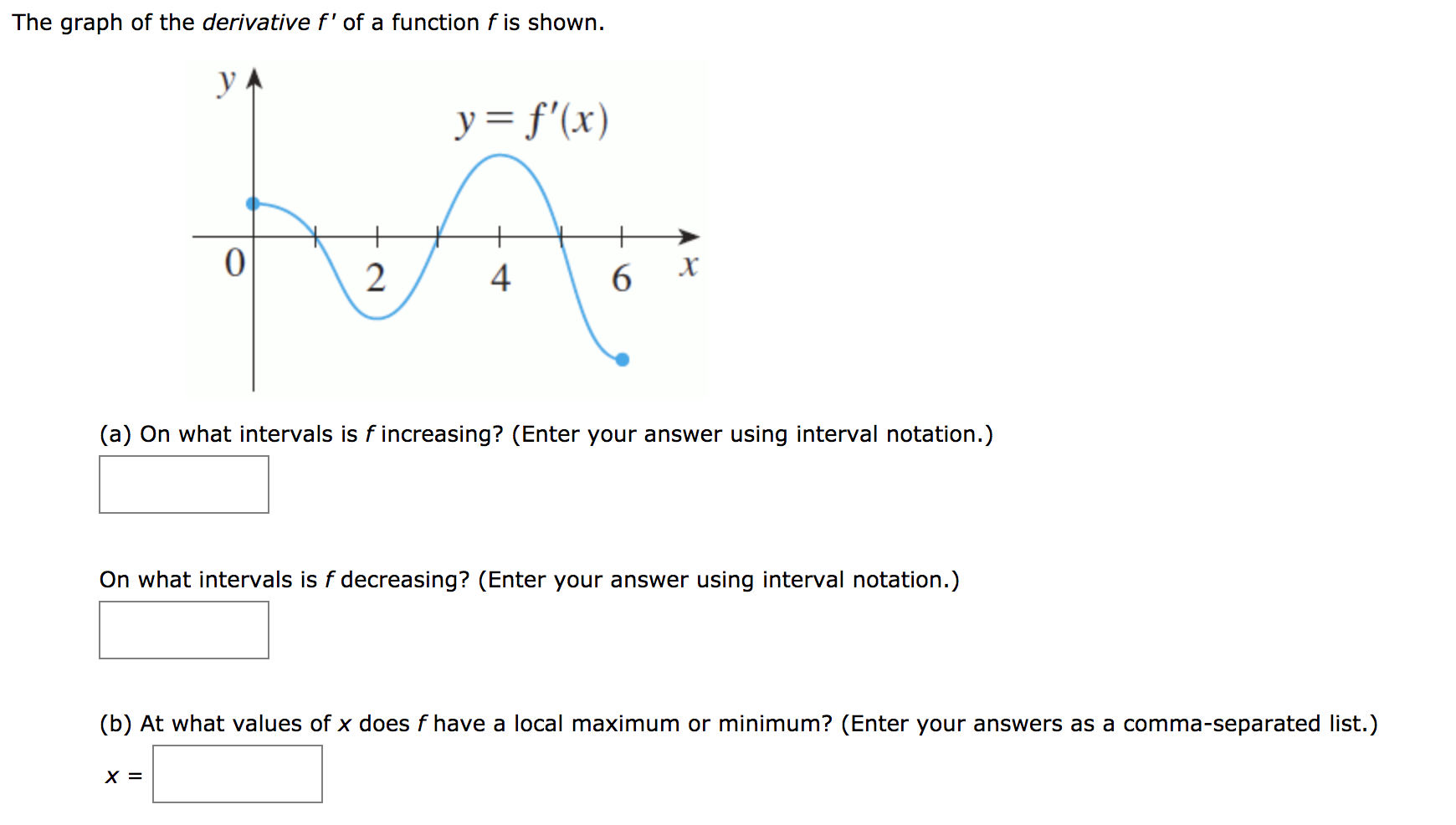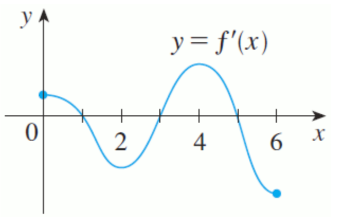# The graph of the derivative f' of a function f is shown.y= f'(x)х(a) On what intervals is f increasing? (Enter your answer using interval notation.)On what intervals is f decreasing? (Enter your answer using interval notation.)(b) At what values of x does f have a local maximum or minimum? (Enter your answers as a comma-separated list.)4.

Question
30 viewshelp_outlineImage TranscriptioncloseThe graph of the derivative f' of a function f is shown. y= f'(x) х (a) On what intervals is f increasing? (Enter your answer using interval notation.) On what intervals is f decreasing? (Enter your answer using interval notation.) (b) At what values of x does f have a local maximum or minimum? (Enter your answers as a comma-separated list.) 4. fullscreen
check_circle

Step 1

The given graph isStep 2

The function f(x) will be increasing where f'(x)>0 so from the graph

Increasing = [0, 1)U(3, 5)

Step 3

The function f(x) will be decreasing where f'(x)<0 so fr...

### Want to see the full answer?

See Solution

#### Want to see this answer and more?

Solutions are written by subject experts who are available 24/7. Questions are typically answered within 1 hour.*

See Solution
*Response times may vary by subject and question.
Tagged in